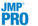Fitting Linear Models > Generalized Linear Models > Overview of the Generalized Linear Model Personality
Publication date: 07/30/2020

# Overview of the Generalized Linear Model Personality

Traditional linear models are used extensively in statistical data analysis. However, there are situations that violate the assumptions of traditional linear models. In these situations, traditional linear models are not appropriate. Traditional linear models assume that the responses are continuous and normally distributed with constant variance across all observations. These assumptions might not be reasonable. For example, these assumptions are not reasonable if you want to model counts, or if the variance of the observed responses increases as the response increases. Another example of violating the assumptions of traditional linear models is when the mean of the response is restricted to a specific range of values, such as proportions that fall between 0 and 1.

For situations such as these that fall into a wider range of data analysis problems, generalized linear models can be applied. Generalized linear models are an extension of traditional linear models. A generalized linear model consists of a linear component, a link function, and a variance function. The link function, g(μi) = xiβ, is a monotonic and differentiable function that describes how the expected value of Yi is related to the linear predictors. An example of generalized linear regression is Poisson regression, where log(μi) is the link function. For a complete list of the generalized linear regression models available using the Generalized Linear Models personality of the Fit Model platform, see Statistical Details for the Generalized Linear Model Personality.

Fitted generalized linear models can be summarized and evaluated using the same statistics as traditional linear models. The Fit Model platform provides parameter estimates, standard errors, goodness-of-fit statistics, confidence intervals, and hypothesis tests for generalized linear models. It should be noted that exact distribution theory is not always available or practical for generalized linear models. Therefore, some inference procedures are based on asymptotic results.

An important aspect of fitting generalized linear models is the selection of explanatory variables in the model. Changes in goodness-of-fit statistics are often used to evaluate the contribution of subsets of explanatory variables to a particular model. The deviance is defined as twice the difference between the maximum attainable value of the log-likelihood function and the value of the log-likelihood function at the maximum likelihood estimates of the regression parameters. The deviance is often used as a measure of goodness of fit. The maximum attainable log-likelihood is achieved with a model that has a parameter for every observation.For variable selection and penalized methods in generalized linear modeling, you can use the Generalized Regression personality of the Fit Model platform in JMP Pro. See Generalized Regression Models.